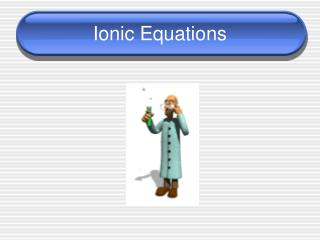DownloadDownload PresentationIonic Equations

# Ionic Equations

Download Presentation## Ionic Equations

- - - - - - - - - - - - - - - - - - - - - - - - - - - E N D - - - - - - - - - - - - - - - - - - - - - - - - - - -
##### Presentation Transcript

1. Ionic Equations

2. Equations • ____________ – show the complete chemical formulas. Does not indicate ionic character • ____________– shows all ions. Actually how the particles exist in the solution

3. Steps for Writing Ionic Equations • Write the balances molecular equation (balanced chemical equation) • Break every thing down into its ions EXCEPT the solid, gas, or water (complete ionic equation) • Cross out everything that is the same on both sides (spectator ions) • Write what is left (net ionic equation)

4. Rules • When writing ionic equations, you must keep together the solid, gas, or water • ____________– ions that appear on both sides of the equation. They have very little to do with the chemical reaction

5. Example • Write the balanced chemical equation, the complete ionic equation, and the net ionic equation for: • lead (II) nitrate and potassium iodide. Solid potassium iodide will be formed.

6. Another Example • Write the balanced chemical equation, complete ionic equation, and net ionic equation for: • calcium chloride and sodium carbonate. Solid calcium carbonate will be formed.

7. What if water is formed? • Write the balanced chemical equation, complete ionic equation, and net ionic equation for: • Calcium hydroxide and nitric acid